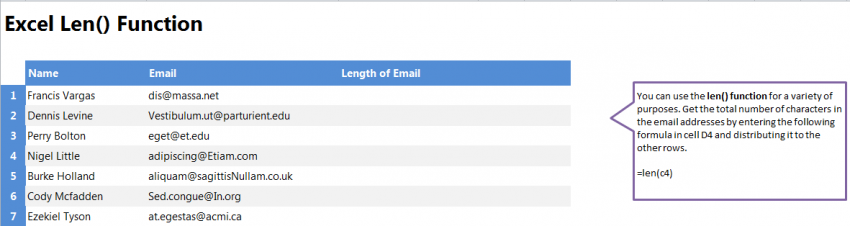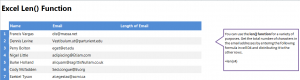# Len Formula20
AprLearn how to use the Excel Len formula in your spreadsheet. The Len function will retrieve how many characters are in a cell or range of cells in your spreadsheet. The formula is easy to use and apply en masse to your entire spreadsheet, and is good when working with large amounts of information.

Download the example Excel spreadsheet and practice this function.

Once you have downloaded the spreadsheet, open it to see three columns: One with names, one with emails, and a blank column for you to practice the formula. This is an example of how a spreadsheet may look when you import the names and emails of a group of people, but the len function will work with virtually any spreadsheet.

Start by selecting cell D4. Type in:

=len(C4)

This is telling Excel to retrieve the amount of characters contained in cell C4. You could also enter a range of cells, but in the example we will start with just each individual email cell.

You don’t have to enter the formula into every cell (you can if you really want to). Instead, grab the corner of D4 and drag the cell down the rest of the D column. It will populate each cell with the formula for it’s C column information. Excel will change the formula automatically in each of the cells to only reference the cell to its left.

Want more Excel? Visit our tutorials.